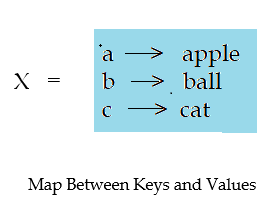# Dictionaries (Creation, Initialization, Access) in Python

Learn: What is Dictionary in Python? In this article, we'll understand the need of dictionariesin python. We'll also discuss about various dictionaries functions and also understand the difference between list and dictionary.
Submitted by Abhishek Jain, on November 29, 2017

A dictionary is a mapping between a set of indices (keys) and a set of values. It is an extremely useful data storage construct where each element is accessed by a unique key.

A dictionary is like a list, just differ in indexing. In a list, an index value is an integer, while in a dictionary index value can be any other data type called keys. It stores and retrieves the key-value pairs, where each value indexed by a unique key.

Syntax:

```Dictionary = {'key1': 'value1','key2': 'value2',...,'keyn': 'valuen'}
```

Example:

```>>> X= {'a' :"apple", 'b' :"ball", 'c' :"cat"}
>>> print X
```

Output

`{'a' :'apple', 'b' :'ball', 'c' :'cat'}`

In the above example, we have created a list where each alphabet maps a English word i.e., keys are in characters (alphabets) and values are in strings.## Basic Operations in Dictionaries

### 1) Creation

We can create a dictionary using built-in function dict(), which creates a new dictionary with no items.We can also create dictionary using {}.

Example

```>>>A=dict()
>>>print A
```

Output

`{}`

Where, {} represents empty string.

### 2) Initialization and Accessing

To initialize or add an item to the dictionary, square brackets with unique keys are used.

Example

```>>> A=dict()
>>> A['a']="apple"
>>> A['b']="ball"
>>> A['c']="cat"
>>> print A
```

Output

`{'a' :'apple' ,'c' :'cat' , 'b' :'ball' }`

Note: May you observe the order of the key-value pairs is not in same order (i.e., input and output orders are not same). Because the order of items in a dictionary is unpredictable.

Similarly, mapped keys to the dictionary items are used under square brackets to access elements of the dictionary.

Example

```>>> A={'oct':"october",'nov':"november",'dec':"december"}
>>> print A['nov']
>>> print A['oct']
```

Output

```november
october
```

### 3) Traversing

Traversing refers to visiting each element index at least one to access its value. This can be done using looping or say by using 'for-loop'.

Example

```>>> A={'a':"apple",'b':"ball",'c':"cat",'d':"doll"}
>>> for i in A:
printi,":",A[i]
```

Output

```a : apple
c : cat
b : ball
d : doll
```

As said previously, the order of items in a dictionary is unpredictable.

Preparation